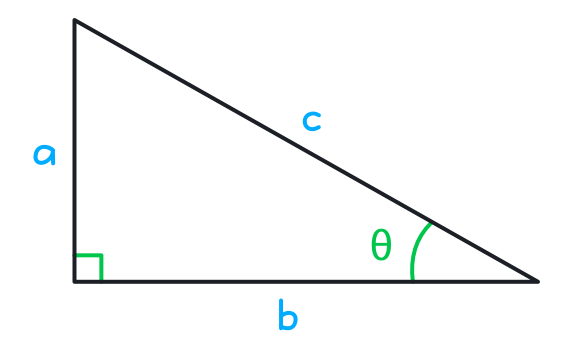### Still have math questions?

Scientific Notation Length Conversion Area Conversion

### Knowledge

Trigonometric functions: (also called circular functions, angle functions) are real functions which relate an angle of a right-angled triangle to ratios of two side lengths.sinθ = ac
cosθ = bc
tanθ = ab
cscθ = ca
secθ = cb
cotθ = ba
##### Relationship

sinθ = cos(π2 - θ) = 1cscθ

cosθ = sin(π2 - θ) = 1secθ

tanθ = sinθ cosθ = cot(π2 - θ) = 1cotθ

cotθ = cosθ sinθ = tan(π2 - θ) = 1tanθ

secθ = csc(π2 - θ) = 1cosθ

cscθ = sec(π2 - θ) = 1sinθ

#### Example

Find the x value of the equation：sin(x)-cos(x)=0

sin(x) - cos(x) = 0
sin(x) = cos(x)
- 2sin(π4 - x) = 0
x = π4+kπ , k ∈ Z
Please provide numbers only. Please provide at least two numbers. Please provide 2 to 10 non-zero integer numbers. None